## How To Calculate or Assign Letter Grade In Excel?

To assign letter grade for each student based on their scores may be a common task for a teacher. For example, I have a grading scale defined where the score 0-59 = F, 60-69 = D, 70-79 = C, 80-89 = B, and 90-100 = A as following screenshot shown. In Excel, how could you calculate letter grade based on the numeric score quickly and easily?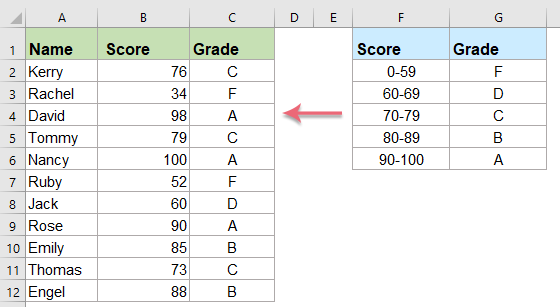#### Calculate letter grade based on score values with IF function

The generic syntax is:

=IF (condition1, value_if_true1, IF (condition2, value_if_true2, IF (condition3, value_if_true3, value_if_false3)))
• condition1, condition2, condition3: The conditions you want to test.
• value_if_true1, value_if_true2, value_if_true3: The value that you want to return if the result of the conditions are TRUE.
• value_if_false3: The value that you want to return if the result of the condition is FALSE.

1. Please enter or copy the below formula into a blank cell where you want to get the result:

=IF(B2>=90,"A",IF(B2>=80,"B",IF(B2>=70,"C",IF(B2>=60,"D","F"))))

Explanation of this complex nested IF formula:

• If the Score (in cell B2) is equal or greater than 90, then the student gets an A.
• If the Score is equal or greater than 80, then the student gets a B.
• If the Score is equal or greater than 70, then the student gets a C..
• If the Score is equal or greater than 60, then the student gets a D.
• Otherwise the student gets an F.

Tips: In the above formula:

• B2: is the cell which you want to convert the number to letter grade.
• the numbers 90, 80, 70, and 60: are the numbers you need to assign the grading scale.

2. Then, drag the fill handle down to the cells to apply this formula, and the letter grade has been displayed in each cell as follows: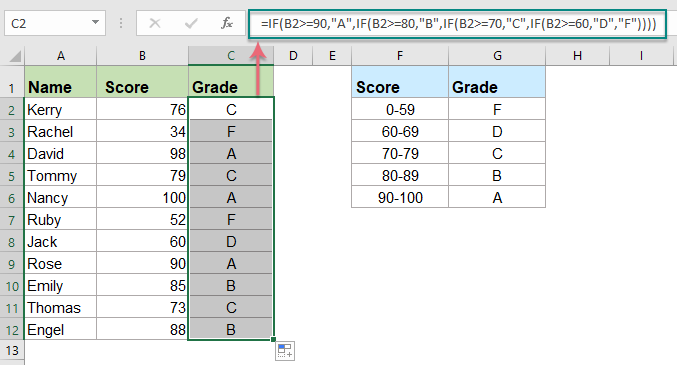Click to know more IF function...## Layoff season is coming, still work slowly? -- Office Tab boosts your pace, saves 50% work time!

•  Amazing! The operation of multiple documents is even more relaxing and convenient than single document;
•  Compared with other web browsers, the interface of Office Tab is more powerful and aesthetic;
•  Reduce thousands of tedious mouse clicks, say goodbye to cervical spondylosis and mouse hand;
•  Be chosen by 90,000 elites and 300+ well-known companies!

#### Calculate letter grade based on score values with VLOOKUP function

If the above nested if function is somewhat difficult for you to understand, here, the Vlookup function in excel also can do you a favor.

The generic syntax is:

=VLOOKUP(lookup_value, table_array, col_index_num, [range_lookup])
• lookup_value: The value that you want to search and find in the table_array.
• table_array: A range of cells in the source table containing the data you want to use.
• col_index_num: The column number in the table_array that you want to return the matched value from.
• range_lookup: A value is either TRUE or FALSE.
• if TRUE or omitted, Vlookup returns either an exact or approximate match
• if FALSE, Vlookup will only find an exact match

1. Firstly, you should create a lookup table as below screenshot shown, and then use the Vlookup function with approximate math to get the result.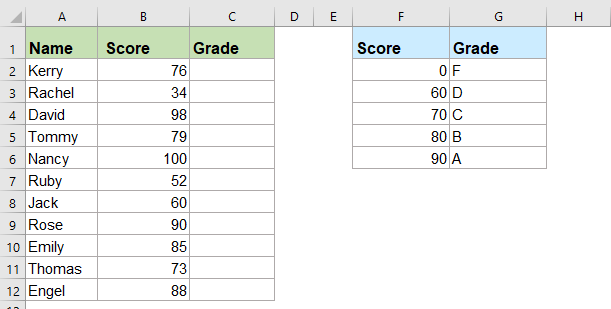Note: It is important that the lookup table must be sorted in ascending order for the VLOOKUP formula to get proper result with an approximate match.

2. Then, enter or copy the following formula into a blank cell – C3, for instance:

=VLOOKUP(B2,\$F\$2:\$G\$6,2,TRUE)

Tips: In the above formula:

• B2: refers to the student score that you want to calculate the letter grade.
• \$F\$2:\$G\$6: It is the table where lookup value will be returned from.
• 2: The column number in the lookup table to return the matched value.
• TRUE: Indicates to find the approximate match value.

3. And then, drag the fill handle down to the cells that you want to apply this formula, now, you can see all the letter grades based on the corresponding grade scale table are calculated at once, see screenshot:Click to know more VLOOKUP function...

#### Calculate letter grade based on score values with IFS function (Excel 2019 and Office 365)

If you have Excel 2019 or Office 365, the new IFS function also can help you to finish this job.

The generic syntax is:

=IFS( logical_test1, value_if_true1, [logical_test2, value_if_true2],... )
• logical_test1: The first condition that evaluates to TRUE or FALSE.
• value_if_true1: Returns the result if logical_test1 is TRUE. It can be empty.
• logical_test2: The second condition that evaluates to TRUE or FALSE.
• value_if_true2: Returns the second result if logical_test2 is TRUE. It can be empty.

1. Please enter or copy the below formula into a blank cell:

=IFS(B2>=90,"A",B2>=80,"B",B2>=70,"C",B2>=60,"D",B2<60,"F")

2. Then, drag the fill handle down to the cells to apply this formula, and the letter grade has been displayed as following screenshot shown: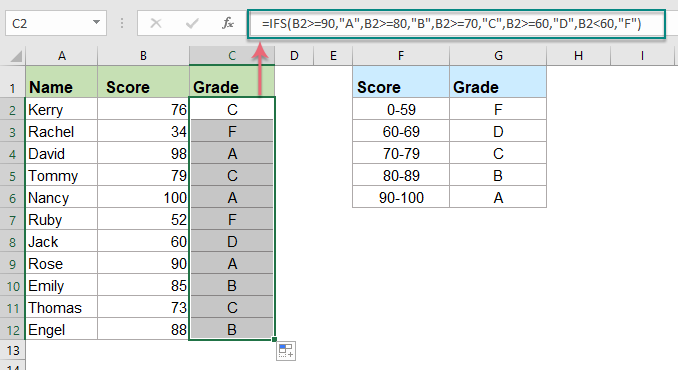Click to know more IFS function...

#### More relative text category articles:

• Categorize Data Based On Values In Excel
• Supposing, you need to categorize a list of data based on values, such as, if data is greater than 90, it will be categorized as High, if is greater than 60 and less than 90, it will be categorized as Medium, if is less than 60, categorized as Low, how could you solve this task in Excel?
• Assign A Value Or Category Based On A Number Range
• This article is talking about assigning value or category related to a specified range in Excel. For example, if the given number is between 0 and 100, then assign value 5, if between 101 and 500, assign 10, and for range 501 to 1000, assign 15. Method in this article can help you get through it.
• Assign Serial Number To Duplicate Or Unique Values
• If you have a list of values which contains some duplicates, is it possible for us to assign sequential number to the duplicate or unique values? It means giving a sequential order for the duplicate values or unique values. This article, I will talk about some simple formulas to help you solving this task in Excel.
• Convert Letter Grade To Number In Excel
• If you have a sheet which contains student names and the letter grades, now you want to convert the letter grades to the relative number grades as below screenshot shown. You can convert them one by one, but it is time-consuming while there are so many to convert.

## The Best Office Productivity Tools

### Kutools for Excel Solves Most of Your Problems, and Increases Your Productivity by 80%

• Super Formula Bar (easily edit multiple lines of text and formula); Reading Layout (easily read and edit large numbers of cells); Paste to Filtered Range...
• Merge Cells/Rows/Columns and Keeping Data; Split Cells Content; Combine Duplicate Rows and Sum/Average... Prevent Duplicate Cells; Compare Ranges...
• Select Duplicate or Unique Rows; Select Blank Rows (all cells are empty); Super Find and Fuzzy Find in Many Workbooks; Random Select...
• Exact Copy Multiple Cells without changing formula reference; Auto Create References to Multiple Sheets; Insert Bullets, Check Boxes and more...
• Favorite and Quickly Insert Formulas, Ranges, Charts and Pictures; Encrypt Cells with password; Create Mailing List and send emails...
• Extract Text, Add Text, Remove by Position, Remove Space; Create and Print Paging Subtotals; Convert Between Cells Content and Comments...
• Super Filter (save and apply filter schemes to other sheets); Advanced Sort by month/week/day, frequency and more; Special Filter by bold, italic...
• Combine Workbooks and WorkSheets; Merge Tables based on key columns; Split Data into Multiple Sheets; Batch Convert xls, xlsx and PDF...
• Pivot Table Grouping by week number, day of week and more... Show Unlocked, Locked Cells by different colors; Highlight Cells That Have Formula/Name...### Office Tab - brings tabbed interface to Office, and make your work much easier

• Enable tabbed editing and reading in Word, Excel, PowerPoint, Publisher, Access, Visio and Project.
• Open and create multiple documents in new tabs of the same window, rather than in new windows.
• Increases your productivity by 50%, and reduces hundreds of mouse clicks for you every day!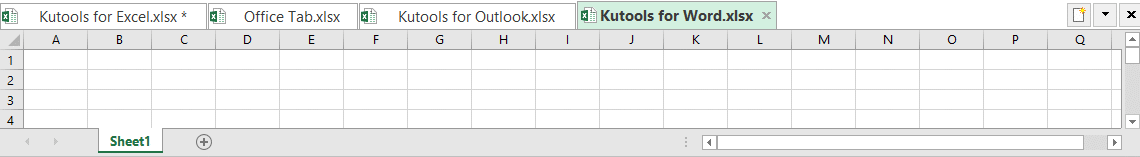No ratings yet. Be the first to rate!
This comment was minimized by the moderator on the site
have learnt the grading system but dont know how to calculate aggregate
This comment was minimized by the moderator on the site
hi pleas help A+ 90 - 100 A 85 - 90- A- 80 - 85 B+ 77 - 80 B 73 - 77 B- 70 - 73 C 60 - 70 F
This comment was minimized by the moderator on the site
RAMOS    PM    PM        AM    7A        AM    AM    N        N    N    N        N    N
HOW TO SUM IF PM=8, AM=8, N=8, 7A=12 in excel. Thank  You very much. This if for our schedule making. thank you!
This comment was minimized by the moderator on the site
12000=300013=350014=400015=500016000 or above=6000
Need Help

This comment was minimized by the moderator on the site
=IF(F2>95,"A+",IF(AND(F2>90,F2<95),"A-",IF(F2>85,"B",IF(AND(F2>80,F2<F2<85),"B-",IF(F2>75,"C",IF(AND(F2>70,F2<75),"C-",IF(F2>65,"D+",IF(AND(F2>60,F2<65),"D-","F")))))))) try this
This comment was minimized by the moderator on the site
Hello yy,Thanks for your support. I slightly changed your formula because it has one error. Please apply the below formula:
=IF(F2>95,"A+",IF(AND(F2>90,F2<95),"A-",IF(F2>85,"B",IF(AND(F2>80,F2<85),"B-",IF(F2>75,"C",IF(AND(F2>70,F2<75),"C-",IF(F2>65,"D+",IF(AND(F2>60,F2<65),"D-","F"))))))))
This comment was minimized by the moderator on the site
=IF(E4<12.5,"D",IF(E4>=12.5,"C",IF(E4>17,"B",IF(E4>22,"B+",IF(E4>29.5,"A",IF(E4>39.5,"A+",IF(E4>44.5,"AA")))))))
This comment was minimized by the moderator on the site
This comment was minimized by the moderator on the site
=IF(E4<12.5,"D",IF(E4>=12.5,"C",IF(E4>17,"B",IF(E4>22,"B+",IF(E4>29.5,"A",IF(E4>39.5,"A+",IF(E4>44.5,"AA")))))))cannot work only grade c & dwhere is error
This comment was minimized by the moderator on the site
please i need help with this
100-80 1
75-79 2
70-74 3
65-69 4
60-64 5
55-59 6
50-54 7
45-49 8
1-44 9
This comment was minimized by the moderator on the site
This comment was minimized by the moderator on the site
Hi,
If
A=2
B=5
C=7
D=10
E=12

How can I count the letter value by excel syntax ?

Thank you.
This comment was minimized by the moderator on the site
Hi, =IFS(F3>90,"A+",F3>85,"A",F3>80,"A-",F3>77,"B+",F3>73,"B",F3>70,"B-",F3>60,"C",F3<60)
I tried to input this and I keep getting this alert "You've entered too few arguments for this function."would you help me please I want my answer as: A+=90-100 A=85-90 A-=80-85 B+=77-70 B=73-77 B-=70-73 C=60-70 F=>60
This comment was minimized by the moderator on the site